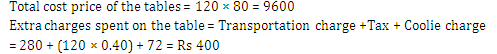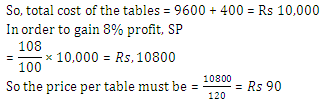# Quantitative MCQ - 15

## 30 Questions MCQ Test Quantitative Aptitude for Competitive Examinations | Quantitative MCQ - 15

Description
This mock test of Quantitative MCQ - 15 for Quant helps you for every Quant entrance exam. This contains 30 Multiple Choice Questions for Quant Quantitative MCQ - 15 (mcq) to study with solutions a complete question bank. The solved questions answers in this Quantitative MCQ - 15 quiz give you a good mix of easy questions and tough questions. Quant students definitely take this Quantitative MCQ - 15 exercise for a better result in the exam. You can find other Quantitative MCQ - 15 extra questions, long questions & short questions for Quant on EduRev as well by searching above.
QUESTION: 1

### Directions (1-5): What should come in place of the question mark (?) in the following questions?  7960+2956-8050+4028= ?

Solution: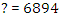QUESTION: 2

### Directions (1-5): What should come in place of the question mark (?) in the following questions?  25×3.25+50.4÷24= ?

Solution: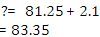QUESTION: 3

### Directions (1-5): What should come in place of the question mark (?) in the following questions?  350% of ? ÷50+248=591

Solution: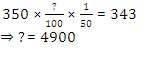QUESTION: 4

Directions (1-5): What should come in place of the question mark (?) in the following questions?

1/2  of 3842+15% of ?=2449

Solution: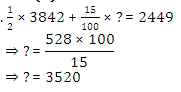QUESTION: 5

Directions (1-5): What should come in place of the question mark (?) in the following questions?

(833.25-384.45)÷24= ?

Solution: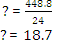QUESTION: 6

Divide Rs. 1301 between A and B, so that the amount of A after 7 years is equal to the amount of B after 9 years, the interest being compounded at 4% per annum.

Solution: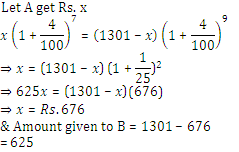QUESTION: 7

Amit borrowed Rs. 800 at 10% rate of interest. He repaid Rs. 400 at the end of first year. What is the amount he is required to pay at the end of second year to discharge his loan which was calculated at compound interest?

Solution: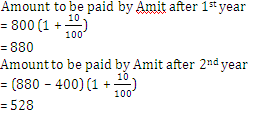QUESTION: 8

A man borrows Rs. 4000 from a bank at 15/2% compound interest. At the end of every year, he pays Rs. 1500 as part repayment of loan and interest. How much does he still owe to the bank after three such instalments?

Solution: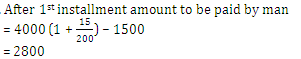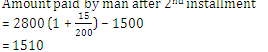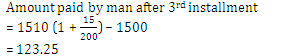QUESTION: 9

The rates of simple interest in two banks A and B are in the ratio 5 : 4. A person wants to deposit his total savings in two banks in such a way that he receives equal half yearly interest from both. He should deposit the savings in banks A and B in the ratio:

Solution: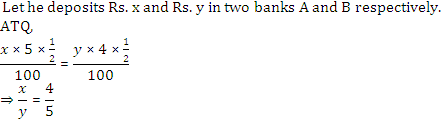QUESTION: 10

An article costing Rs. 9,000 is sold at a discount which is equal to the simple interest on Rs. 3,000 for N months. Find N if the rate of discount is same as the rate of interest.

Solution: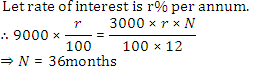QUESTION: 11

There is a sequence of 11 consecutive odd numbers. If the average of first 7 numbers is X, then find the average of all the 11 integers.

Solution: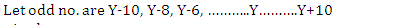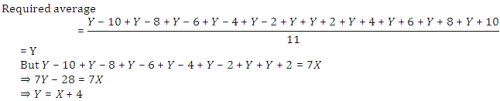QUESTION: 12

A milkman buys milk contained in 10 vessels of equal size. If he sells his milk at Rs. 5 a litre, he loses Rs. 200; if he sells it at Rs. 6 a litre, he would gain Rs. 150 on the whole. Find the number of litres contained in each vessel:

Solution: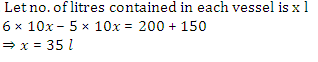QUESTION: 13

The mean of 25 observations was found to be 78.4. But later on it was found that 96 was misread as 69. The correct mean is:

Solution: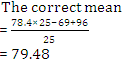QUESTION: 14

A trader has 50 kg of rice, a part of which he sells at 10% profit and the rest at 5% loss. He gains 7% on the whole. How much was sold at 10% gain and how much was sold at 5% loss?

Solution: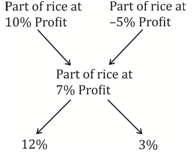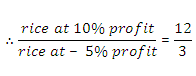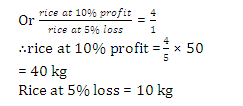QUESTION: 15

By selling 12 marbles for a rupee, a shopkeeper loses 20% In order to gain 20% in the transaction, he should sell the marbles at the rate of how many marbles for a rupee?

Solution: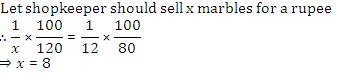QUESTION: 16

Directions (Q.16-20): What approximate value will come in place of question mark (?) in the following questions? (You are not expected to calculate the exact value.)

(7171+3854+1195)÷(892+214+543)=?

Solution: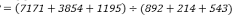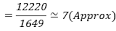QUESTION: 17

Directions (Q.16-20): What approximate value will come in place of question mark (?) in the following questions? (You are not expected to calculate the exact value.)

69.008% of 699.998+32.99% of 399.999= ?

Solution: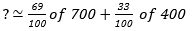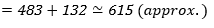QUESTION: 18

Directions (Q.16-20): What approximate value will come in place of question mark (?) in the following questions? (You are not expected to calculate the exact value.)

( 24.99 )2 + ( 31.05 )2 = (?)2

Solution: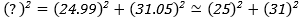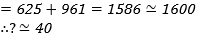QUESTION: 19

Directions (Q.16-20): What approximate value will come in place of question mark (?) in the following questions? (You are not expected to calculate the exact value.)

115% of 624+   2/7    of 419= ?

Solution: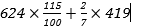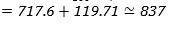QUESTION: 20

Directions (Q.16-20): What approximate value will come in place of question mark (?) in the following questions? (You are not expected to calculate the exact value.)

9654÷21+7638÷44= ?

Solution: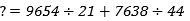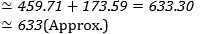QUESTION: 21

Directions (Q.21-25): In the following number series only one number is wrong. Find out wrong number.

71,  90, 128, 185, 261, 365

Solution: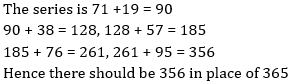QUESTION: 22

Directions (Q.21-25): In the following number series only one number is wrong. Find out wrong number.

6, 7, 16, 41, 90, 154, 292

Solution: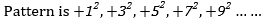QUESTION: 23

Directions (Q.21-25): In the following number series only one number is wrong. Find out wrong number.

4, 2.5,    3.5, 6.5, 15.5, 41.25, 126.75

Solution: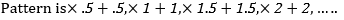QUESTION: 24

Directions (Q.21-25): In the following number series only one number is wrong. Find out wrong number.

5,    7, 16, 57, 244, 1245, 7506

Solution: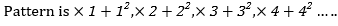QUESTION: 25

Directions (Q.21-25): In the following number series only one number is wrong. Find out wrong number.

1,       4,       25,       256,       3125,       46656,       823543

Solution: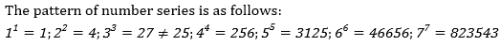QUESTION: 26

Weight of two persons A and B are in the ratio of 3 : 5. A’s weight increases by 20% and the total weight of A and B together becomes 80 kg, with an increase of 25%. By what percent did the weight of B increase?

Solution: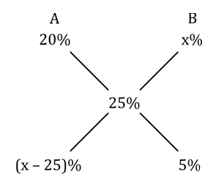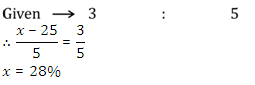QUESTION: 27

The ratio of the prices of two houses A and B was 4 : 5 last year. This year, the price of A is increased by 25 percent and that of B by Rs. 50,000. If their prices are now in the ratio 9 : 10, the price of A last year was:

Solution: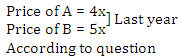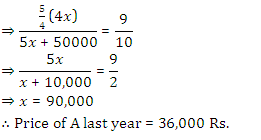= 360000

QUESTION: 28

A man borrows Rs. 6000 at 10% compound rate of interest. He pays back Rs. 2000 at the end of each year to clear his debt. The amount that he should pay to clear all his dues at the end of third year is:

Solution: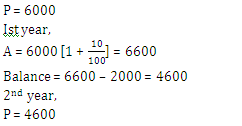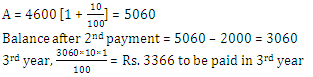QUESTION: 29

A vessel contains a mixture of liquid A and liquid B in the ratio 3 : 2. Five litres of mixture are taken out and replaced by liquid B. Thereafter, the ratio of liquid A and liquid B becomes 2 : 3. Find the quantity of liquid A in the mixture initially.

Solution: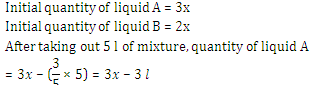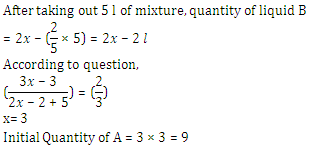QUESTION: 30

A man purchased 120 laptop tables at the rate of Rs. 80 per table. He spent Rs 280 on transportation, paid tax at the rate of 40 paise per table and paid Rs 72 to the coolie. If he wants to have a gain of 8%, the selling price per table must be (in Rs)

Solution: